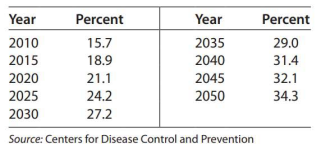# Modeling Projections indicate that the percent of U.S. adults with diabetes will dramatically increase. The table gives the percent of U.S. adults with diabetes for selected years from 2010 and projected to 2050. Find a logarithmic model, y = f ( x ) , for these data. Use x as the number of years after 2000. Find the function that models the rate of change of the percent of U.S. adults with diabetes. Find and interpret f ( 25 ) and f ' ( 25 ) .### Mathematical Applications for the ...

11th Edition
Ronald J. Harshbarger + 1 other
Publisher: Cengage Learning
ISBN: 9781305108042

#### Solutions

Chapter
Section### Mathematical Applications for the ...

11th Edition
Ronald J. Harshbarger + 1 other
Publisher: Cengage Learning
ISBN: 9781305108042
Chapter 11, Problem 18T
Textbook Problem
1 views

## Modeling Projections indicate that the percent of U.S. adults with diabetes will dramatically increase. The table gives the percent of U.S. adults with diabetes for selected years from 2010 and projected to 2050. Find a logarithmic model, y = f ( x ) , for these data. Use x as the number of years after 2000. Find the function that models the rate of change of the percent of U.S. adults with diabetes. Find and interpret f ( 25 ) and f ' ( 25 ) .This textbook solution is under construction.

### Still sussing out bartleby?

Check out a sample textbook solution.

See a sample solution

#### The Solution to Your Study Problems

Bartleby provides explanations to thousands of textbook problems written by our experts, many with advanced degrees!

Get Started